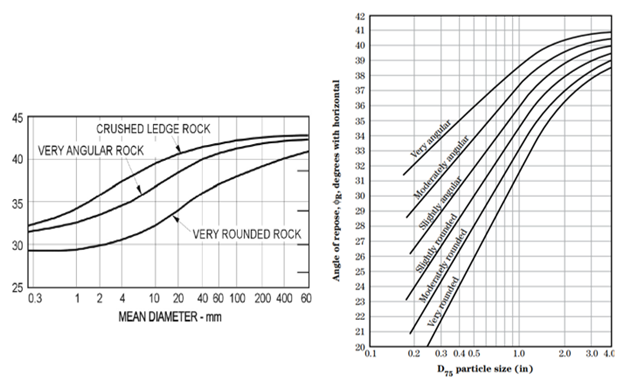The Riprap Calculator requires five additional measurements and parameters that do not come directly from HEC-RAS results. Three of those parameters populate with default values, two require user input: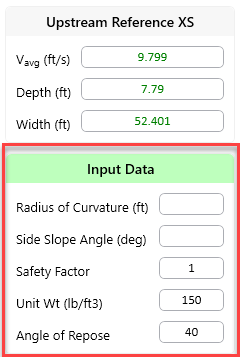# Radius of Curvature (R)

Both the riprap and scour equations are based on a dimensionless ratio of the radius of curvature to the channel width (R/W) which is a relative measure of bend severity. HEC-RAS will compute the width of the selected cross section. The numerical width is the wetted top-width between the channel banks (or the distance between the channel banks if the entire channel is inundated).

You must compute and enter a Radius of Curvature which quantifies the severity of a bend.

An example of the Jones Creek Bend on the Brazos River in Fort Bend County, Texas is included below. A circle was fit to the bend in Google Earth, which reported the radius. This bend has a radius of curvature of 1,155 ft and a channel width of 410 ft for an R/W ratio of 2.8. The riprap and bend scour calculations are all very sensitive to the R/W ratio. Therefore, identifying the appropriate radius of curvature, and carefully distinguishing the curvature scales of compound bends (see next section) will affect results.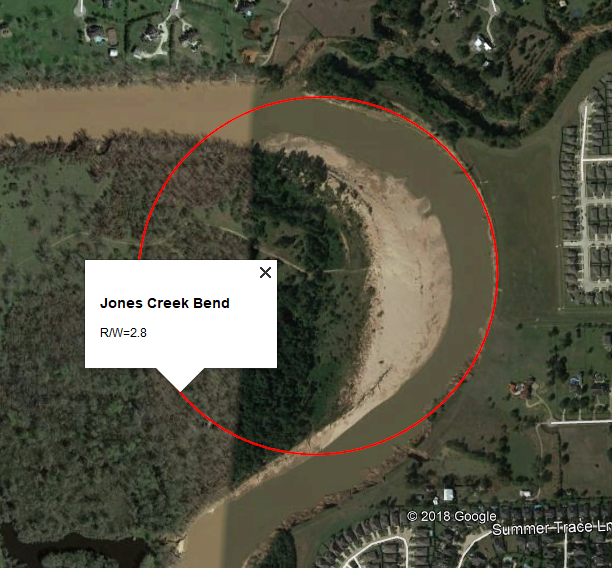Modeling Note: Radius of Curvature Extent

Different agencies and manuals of practice measure the radius of curvature differently.  Some extend the circle that measures this radius to the edge of the bank.  Others extend it to the centerline of the river.  This variability in practice is part of the uncertainty in these calculations.

## Computing the Radius of Curvature

You can compute the radius of curvature in a GIS, but Google Earth also has all the tools you will need to measure this input. The Radius of Curvature computation has three steps:

• Delineate the critical bankline, which is usually on the outside of the downstream third of the bend.
• Fit a circle to the critical section of the bend where the bend scour equation will be applied.
• Compute the radius of that circle.

The following figure illustrates an example radius of curvature calculation.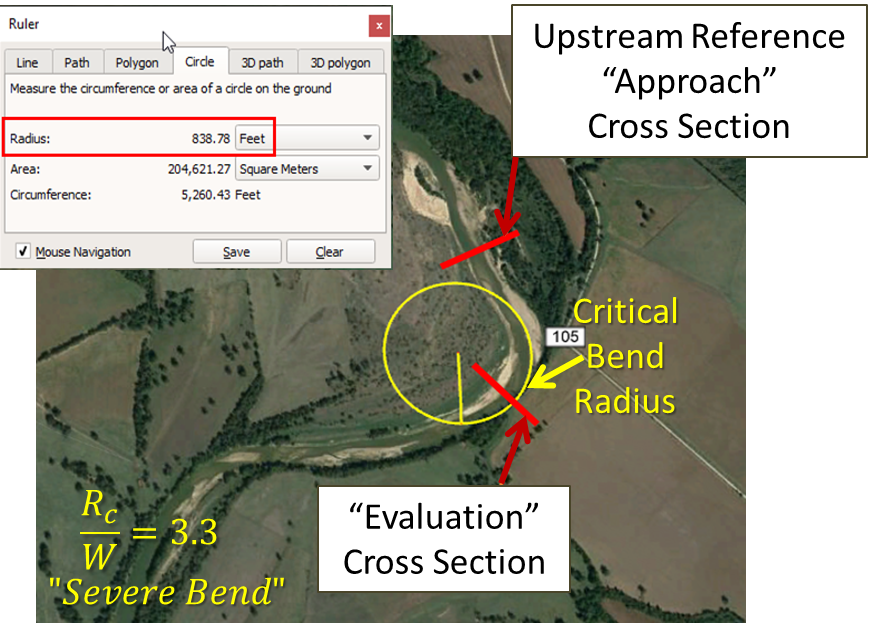## Compound Bends

Some bends do not have simple geometries, making it difficult to fit one, idealized circle to the bend. Bends that curve on multiple scales are called "compound bends." Brice (1974) Brice, J. (1974) "Evolution of Meander Loops" Geological Society of America Bulletin, 85, 581-586. illustrated and classified several examples of compound bends in the figure below:The following figure includes an example of a compound section, which includes river bends at multiple scales (David May, personal communication). It is important to select the bend radius (and scour) at the critical location on a compound bend and the critical (usually the smallest), associated radius of curvature.Radii of curvature appropriate for bend scour analysis on different locations along a compound bend. The section most susceptible to scour is often the downstream, outside bank of a tight sub-meander (the critical radius in the image).
It can be useful to compute stable rock size and scour at several locations within a compound bend to identify the critical location.

For more radius of curvature guidance and examples, see the detailed discussion in the National Cooperative Highway Research Program (2004) Handbook for Predicting Stream Meander Migration and Supporting Software.

# Side Slope ()

There are three common and interchangeable ways to define the side slope angle in engineering and architecture. HEC-RAS usually uses the ratio of horizonal distance to vertical rise (H:V) or the inverse. The Riprap Calculator requires the bank side slope in degrees.  Equations for converting V:H or % slope measurements to degrees (and back again) are included in the next section.

## Converting Side Slope (Degrees, V:H, Percent)

Engineering and architecture use three interchangeable methods to quantify slope: degrees, V:H, and percent. The following equations and table will help you convert between them.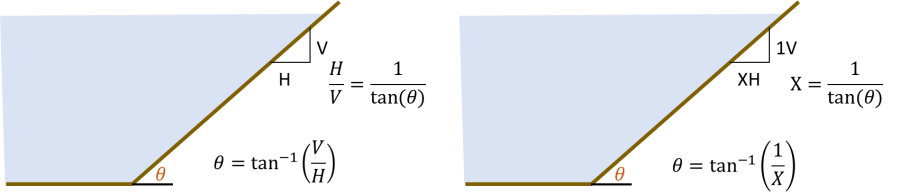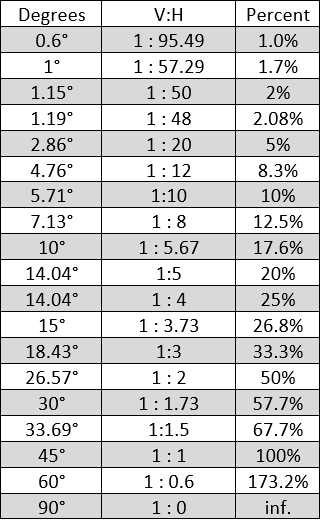# Safety Factor (FS)

HEC-RAS sets the safety factor to 1, by default. This provides the unfiltered results of the equation, and allows the user to make their own choice about the risk tolerance built into the Factor of Safety.

However, USACE Guidance (EM 1110-2-1601) recommends setting the Factor of Safety to 1.1.

The riprap calculator will multiply the calculated d30 by the factor of safety (so FS=1.14 will increase the d30 by 14%), increasing the W30 by approximately 50%.  EM 1110-2-1601 includes several considerations that should lead a project team to increase the Factor of Safety.

# Unit Weight

HEC-RAS uses the unit weight to convert riprap diameter to dry placement weight, using the equation: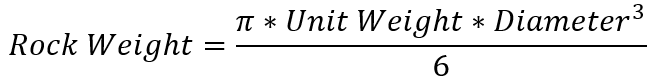This is only used if the user requests results and gradations in terms of weight, instead of rock size (which is a common convention in riprap design).Modeling Note: Spherical Particles

The weight-diameter conversion assumes spherical particles.  The volume is approximately half of an equivalent cube with edges matching the diameter. Some methods suggest using a ratio of 75%-85% of the equivalent volume of a cube, which would increase the weight or a stable particle diameter. Applying the assumption of angular riprap in the calculations does not affect the weight-diameter relationship.

# Angle of Repose (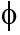)

The angle of repose is the angle of the maximum slope an aggerate develops before it starts to move. It is a function of the size, compaction, and angularity of the material. Riprap tends to vary between 35 and 45 degrees. HEC-RAS populates a default of 40o.The riprap calculation only uses the angle of repose in the analytical calculation of K1.